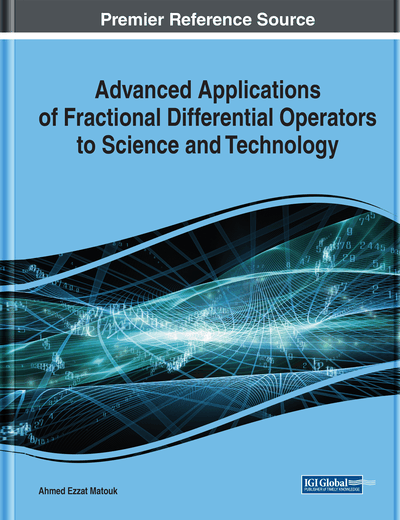# On the Approximate Solution of Caputo-Riesz-Feller Fractional Diffusion Equation

Samir Shamseldeen, Ahmed Elsaid, Seham Madkour
DOI: 10.4018/978-1-7998-3122-8.ch010
OnDemand:
(Individual Chapters)
Available
\$29.50
No Current Special Offers

## Abstract

In this work, a space-time fractional diffusion equation with spatial Riesz-Feller fractional derivative and Caputo fractional time derivative is introduced. The continuation of the solution of this fractional equation to the solution of the corresponding integer order equation is proved. Also, a very useful Riesz-Feller fractional derivative is proved; the property is essential in applying iterative methods specially for complex exponential and/or real trigonometric functions. The analytic series solution of the problem is obtained via the optimal homotopy analysis method (OHAM). Numerical simulations are presented to validate the method and to highlight the effect of changing the fractional derivative parameters on the behavior of the obtained solutions. The results in this work are originally extracted from the author's work.
Chapter Preview
Top

## Introduction

Fractional derivatives have been used for building and invistigating numerous models and systems in various branches of applied sciences. For example, in describing physical models (Al-khedhairi, Matouk, & Khan, 2019); (El-Sayed A. M., 1996); (Shamseldeen, 2018), in biological systems (Elsadany & Matouk, 2015) (Al-Khedhairi, Askar, Matouk, Elsadany, & Ghazel, 2018) (Matouk & Elsadany, 2016), in economic and finance (Khana & Kumar, 2016) (Hegazi, Ahmed, & Matouk, 2013), in engineering systems (El-Sayed, Nour, Elsaid, Matouk, & Elsonbaty, 2016) and in some interdisciplinary fields of science (Bagley & Calico, 1991) (Kader, Latif, & Nour, 2017) (Abdel Latif, El-Shazly, Baleanu, Elsaid, & Nour, 2018) (Shamseldeen, Elsaid, & Madkour, 2019a) (Shamseldeen, Elsaid, & Madkour, 2019b) (Taghavian & Tavazoei, 2019) (Taghavian & Tavazoei, 2018).

Anomalous diffusion is one process that has been successfully modeled by fractional derivatives. This type of diffusion is characterized by the nonlinear dependence of the mean square displacement x(t) of a diffusing particle over time t: x2(t)∝kαtα and it can be interpreted as the Lévy stable densities. On the other hand, in the case of classical diffusion, linear dependence x2(t)∝kt occurs and it follows Gaussian statistics and Fick’s second law for running processes at time t (Metzler & Klafter, 2000). Anomalous diffusion is described by fractional partial differential equations (FPDEs) by replacing classical derivatives with derivatives of fractional order. A type of anomalous diffusion that received great attention is the fractional diffusion equation with spatial Riesz and Riesz-Feller fractional derivatives (Elsaid, 2011)- (Gorenflo, Mainardi, Moretti, Pagnini, & Paradisi, 2002). These fractional derivative operators are used to model phenomena as anomalous diffusion (Metzler & Klafter, 2000), discrete random walk models (Gorenflo, Mainardi, Moretti, Pagnini, & Paradisi, 2002)- (Gorenflo & Vivoli, 2003), and continuous random walk models (Gorenflo & Mainardi, 2003) in the form of FPDEs.

General solutions are obtained for some classes of linear FPDEs, using integral transforms. For example, in (Agrawal, 2002) a general solution is given for a time fractional diffusion-wave equation defined in a bounded space domain. The fractional time derivative is described in the Caputo sense. The finite sine Laplace transform technique is used to obtain the solution. In (Jiang, Liu, Turner, & Burrage, 2012) analytic techniques are introduced to solve certain types of multi-term time–space Caputo–Riesz fractional advection–diffusion equations on a finite domain using the equivalent relationship between the Laplacian operator and the Riesz fractional derivative. In (Mainardi, Pagnini, & Luchko, 2007) a fundamental solution is investigated for the space-time fractional diffusion-wave equation, which is obtained from the standard diffusion equation by replacing the second-order space derivative with a Riesz-Feller derivative and the first-order time derivative with a Caputo derivative. In (Haubold, Mathai, & Saxena, 2011) a solution of a unified reaction–diffusion equation with the Riemann–Liouville fractional derivative as the time-derivative and Riesz–Feller derivative as the space-derivative in terms of H-function. In (Garg, Sharma, & Manohar, 2013) a closed form solution in terms of Mittag-Leffler function is obtained for a space-time fractional telegraph equation with Riesz–Feller derivative as the space-derivative. In (Huang & Liu, 2005) a solution of a space-time fractional advection-dispersion equation is derived in terms of the Green functions.

## Complete Chapter List

Search this Book:
Reset